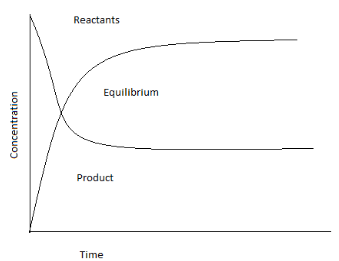Courses
Courses for Kids
Free study material
Offline Centres
MoreLast updated date: 03rd Dec 2023
Total views: 278.7k
Views today: 3.78k

# If the value of $\Delta {{G}^{\circ }}$ for the equilibrium reaction at $300K\text{ is }57.44kJmo{{l}^{-1}}$ , the equilibrium constant ${{K}_{C}}$ is:Verified
278.7k+ views
Hint : To solve this question, try to find two equations of the Gibbs energy change. One reaction should relate △ G with the equilibrium constant and the other should relate to ${{E}_{0}}$ of the cell. Now equate these two and find the answer. Gibbs free energy, denoted $\Delta G$ , combines enthalpy and entropy into one value

Equilibrium in a reaction is a state in which both reactants and products are present in a concentration where no further changes can occur with time and no change in the properties can be observed. In other words, when the rate of forward reaction and reverse reaction are equal, the state of chemical equilibrium is achieved. If $\Delta G$ is positive, then the reaction is nonspontaneous (i.e., the input of external energy is critical for the reaction to occur) and if it's negative, then it's spontaneous (occurs without external energy input). Spontaneous - could be a reaction that's fancy to be natural because it's a reaction that happens by itself with no external action towards it. Non spontaneous - needs constant external energy applied to that so as for the method to continue and once you stop the external action the method will cease. When solving for the equation, if change of G is negative, then we say that the reaction is spontaneous. If the change of G is positive, then it is non-spontaneous. The symbol that's commonly used at no cost ENERGY is G. is more properly considered as "standard free energy change".
To find the equilibrium constant ${{K}_{C}}$ , we will be using the formula $\Delta {{G}^{\circ }}=-RT\ln {{K}_{C}}$ since the reaction is in equilibrium.
Where,
$\Delta {{G}^{\circ }}$ $=$ equilibrium of spontaneity.
$R=$ gas constant found in ideal gas law ( $R=8.314Jmo{{l}^{-1}}{{K}^{-1}}$ )
Now, let’s implement the formula:
$\text{ }\Delta {{G}^{\circ }}=-RT\ln K$
$\Rightarrow 57.44\times {{10}^{3}}=-8.314\times 300\times \ln {{K}_{C}}$ (Substituting the values of $\Delta {{G}^{\circ }}$ , $R$ and $T$ )
$\therefore \ln {{K}_{C}}=-23.029$
Now, taking ${{e}^{x}}$ function: ${{e}^{-23.029}}={{K}_{C}}$
$\therefore {{K}_{C}}=9.968\times {{10}^{-11}}$
Therefore, the value of the equilibrium constant, ${{K}_{C}}$ is $9.968\times {{10}^{-11}}$ .Note :
The number of reactant molecules converting into products and the number of products converting into reactants are the same and this can be carried out anywhere with similar conditions with continuous interchanging of molecules. There are two types of chemical equilibrium: homogeneous equilibrium, where the reactants and the products are in the same phase, and heterogeneous equilibrium, where the reactant and the products are in different phases.